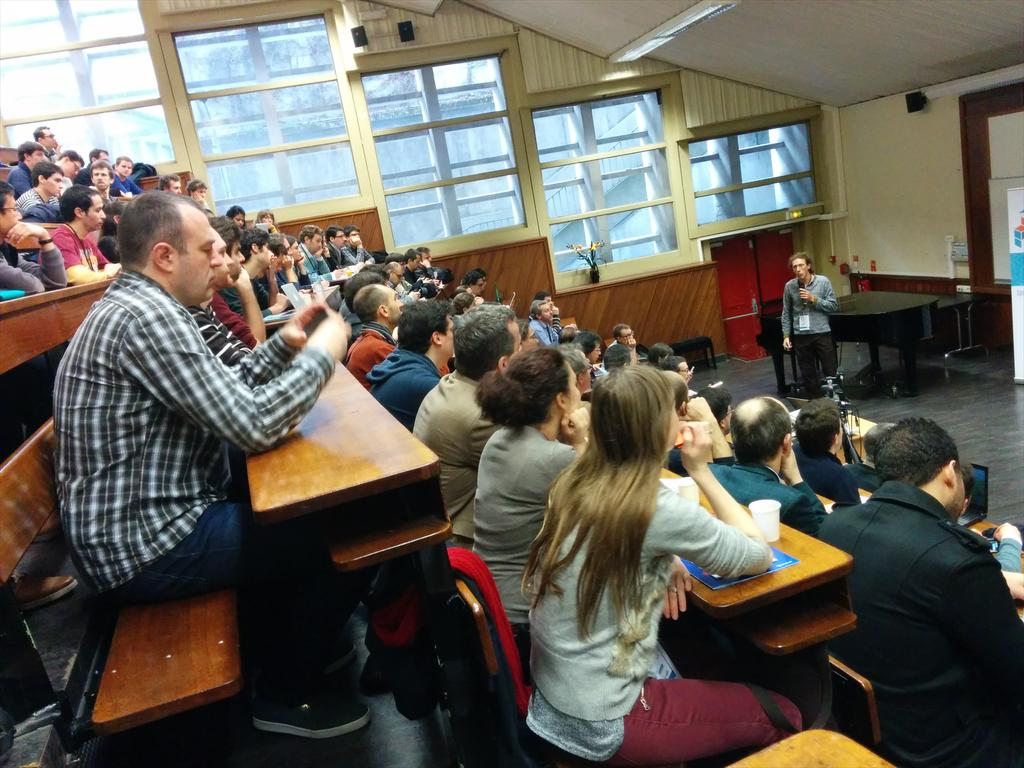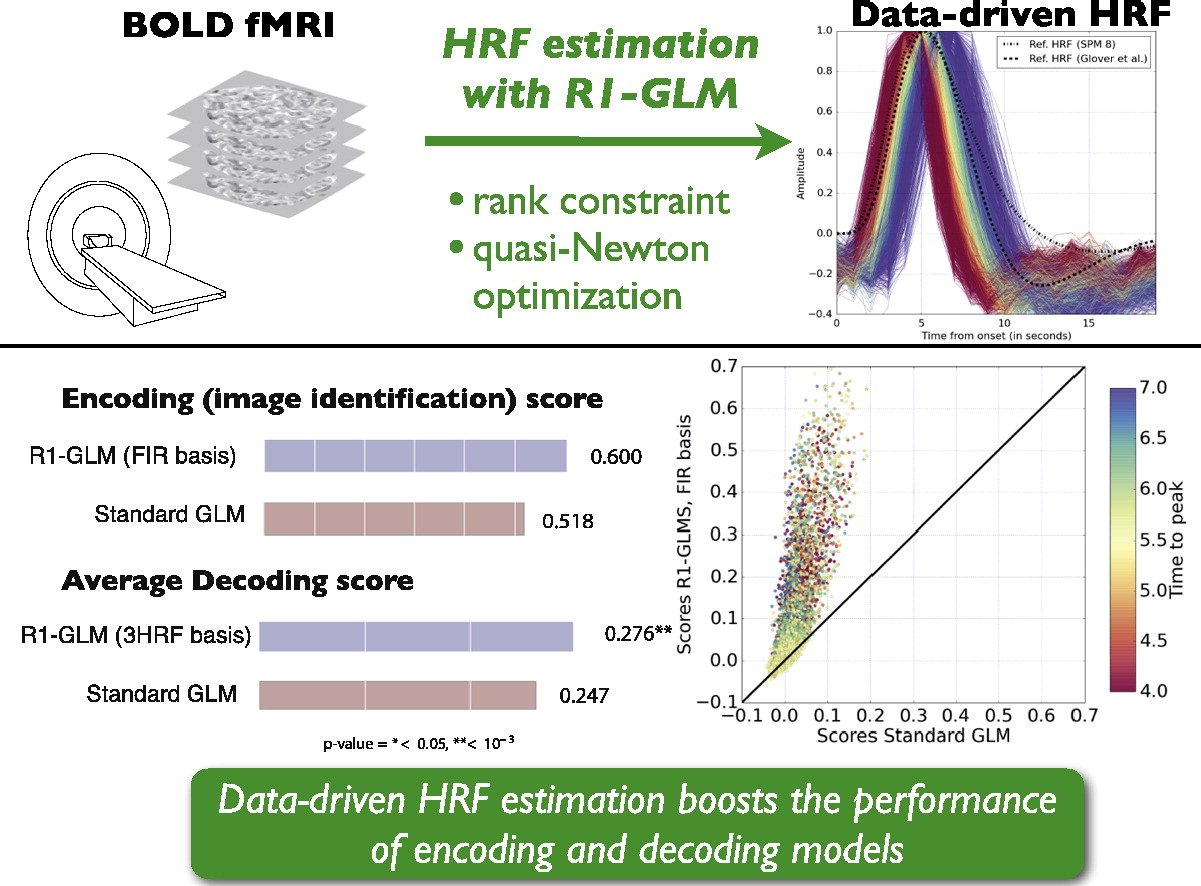Recently I've implemented, together with Arnaud Rachez, the SAGA algorithm in the lightning machine learning library (which by the way, has been recently moved to the new scikit-learn-contrib project). The lightning library uses the same API as scikit-learn but is particularly adapted to online learning. As for the SAGA …

Cross-validation iterators in scikit-learn are simply generator objects, that is, Python objects that implement the __iter__ method and that for each call to this method return (or more precisely, yield) the indices or a boolean mask for the train and test set. Hence, implementing new cross-validation iterators that behave as …

Due to lack of time and interest, I'm no longer maintaining this project. Feel free to grab the sources from https://github.com/fabianp/nbgallery and fork the project.

TL;DR I created a gallery for IPython/Jupyter notebooks. Check it out :-)A couple of months ago I put online …

Last Friday was PyData Paris, in words of the organizers, ''a gathering of users and developers of data analysis tools in Python''.The organizers did a great job in putting together and the event started already with a full room for Gael's keynoteMy take-away message from the talks is …

My latest research paper deals with the estimation of the hemodynamic response function (HRF) from fMRI data.This is an important topic since the knowledge of a hemodynamic response function is what makes it possible to extract the brain activation maps that are used in most of the impressive …

:og_image: http://fa.bianp.net/blog/images/2014/mprof_example.png

One of the lesser known features of the memory_profiler package is its ability to plot memory consumption as a function of time. This was implemented by my friend Philippe Gervais, previously a colleague at INRIA and now at Google.

With …

TL; DR These are some notes on calibration of surrogate loss functions in the context of machine learning. But mostly it is …

As part of the development of memory_profiler I've tried several ways to get memory usage of a program from within Python. In this post I'll describe the different alternatives I've tested.

### The psutil library

psutil is a python library that provides an interface for retrieving information on running processes. It …

In this post I compar several implementations of Logistic Regression. The task was to implement a Logistic Regression model using standard optimization …

TL;DR: I've implemented a logistic ordinal regression or proportional odds model. Here is the Python code

The logistic ordinal regression model …

My latest contribution for scikit-learn is an implementation of the isotonic regression model that I coded with Nelle Varoquaux and Alexandre Gramfort …

Householder matrices are square matrices of the form

$$P = I - \beta v v^T$$

where $\beta$ is a scalar and $v$ is …

Note: this post contains a fair amount of LaTeX, if you don't visualize the math correctly come to its original location

In …

Besides performing a line-by-line analysis of memory consumption, memory_profiler exposes some functions that allow to retrieve the memory consumption of a function in real-time, allowing e.g. to visualize the memory consumption of a given function over time.

The function to be used is memory_usage. The first argument specifies what …

SciPy contains two methods to compute the singular value decomposition (SVD) of a matrix: scipy.linalg.svd and scipy.sparse.linalg.svds. In this post I'll compare both methods for the task of computing the full SVD of a large dense matrix.

The first method, scipy.linalg.svd, is perhaps …

This tutorial introduces the concept of pairwise preference used in most ranking problems. I'll use scikit-learn and for learning and matplotlib for …

My newest project is a Python library for monitoring memory consumption of arbitrary process, and one of its most useful features is the line-by-line analysis of memory usage for Python code. I wrote a basic prototype six months ago after being surprised by the lack of related tools. I wanted …

A little experiment to see what low rank approximation looks like. These are the best rank-k approximations (in the Frobenius norm) to the a natural image for increasing values of k and an original image of rank 512.Python code can be found here. GIF animation made using ImageMagic's convert …

In scipy's development version there's a new function closely related to the QR-decomposition of a matrix and to the least-squares solution of a linear system. What this function does is to compute the QR-decomposition of a matrix and then multiply the resulting orthogonal factor by another arbitrary matrix. In pseudocode …

As a warm-up for the upcoming EuroScipy-conference, some of the scikit-learn developers decided to gather and work together for a couple of days. Today was the first day and there was only a handfull of us, as the real kickoff is expected tomorrow. Some interesting coding happened, although most of …

Ridge coefficients for multiple values of the regularization parameter can be elegantly computed by updating the thin SVD decomposition of the design matrix:

import numpy as np
from scipy import linalg
def ridge(A, b, alphas):
"""
Return coefficients for regularized least squares

min ||A x - b||^2 + alpha ||x||^2 …

Update: a fast and stable norm was added to scipy.linalg in August 2011 and will be available in scipy 0.10 Last week I discussed with Gael how we should compute the euclidean norm of a vector a using SciPy. Two approaches suggest themselves, either calling scipy.linalg.norm …

I was last weekend in FOSDEM presenting scikits.learn (here are the slides I used at the Data Analytics Devroom). Kudos to Olivier Grisel and all the people who organized such a fun and authentic meeting!I've been working lately with Alexandre Gramfort coding the LARS algorithm in scikits.learn. This algorithm computes the solution to several general linear models used in machine learning: LAR, Lasso, Elasticnet and Forward Stagewise. Unlike the implementation by coordinate descent, the LARS algorithm gives the full coefficient path along the …

En la ciudad fabrican a las personas en serie. En el campo, los siguen haciendo a mano: los moldean, los secan al sol y luego los pintan, por eso son tan especiales. Primer concierto de la nueva gira en Mengíbar, y uno de los fines de semana más intensos que …